# Parameters of JFET or Specifications of JFET

During purchasing a JFET for a particular application we need to check the specifications of the device. These specifications are provided by manufacturers. The followings are the parameters used to specify a JFET and these are

## Gate Cut Off Voltage

At a fixed drain voltage, the drain current (ID) of a JFET depends on the gate to source voltage (VGS). If the gate to source voltage decreases from zero in n channel JFET, the drain current also gets decreased accordingly. The relation between gate to source voltage and drain current is given below.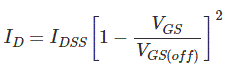After a certain gate to source voltage (VGS), the drain current ID becomes zero. This voltage is known as Cut Off Gate Voltage (VGS(off)). This voltage numerically equals the pinch-off drain to source voltage (Vp). In the case of p channel JFET if we increase the gate terminal voltage from zero the drain current gets decreased and after a certain gate to source voltage, the drain current becomes zero. This voltage is the cut off gate voltage for p channel JFET. It is the gate cut off voltage for p channel JFET.

## Shorted Gate Drain Current

When the gate terminal is grounded (VGS = 0) and positive drain to source voltage (VDS) is being increased slowly in case of n channel JFET, the drain current gets increased linearly. But after pinch-off voltage (Vp), the drain current would not be increased further and gets a constant value. This is the maximum drain current that can flow through the channel when the gate terminal is in ground potential. This current is fixed for a JFET and this is called shorted gated drain current and generally denoted by IDSS.

## Transconductance

Transconductance is the ratio of change in drain current (δID) to change in the gate to source voltage (δVGS) at a constant drain to source voltage (VDS = Constant).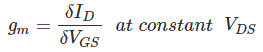This value is maximum at VGS = 0. This is denoted by gmo. This maximum value (gmo) is specified in a JFET data sheet. The transconductance at any other value of gate to source voltage (gm) can be determined as follows. The expression of drain current (ID) isBy partial differentiating the expression of drain current (ID) in respect of gate to source voltage (VGS)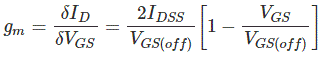At VGS = 0, the transconductance gets its maximum value and that is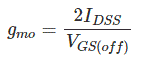Therefore, we can write,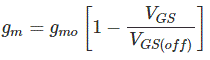## Dynamic Output Resistance

This is the ratio of change of drain to source voltage (δVDS) to the change of drain current (δID) at a constant gate to source voltage (VGS = Constant). The ratio is denoted as rd.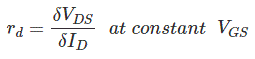## Amplification Factor

The amplification factor is defined as the ratio of change of drain voltage (δVDS) to change of gate voltage (δVGS) at a constant drain current (ID = Constant).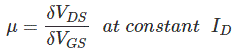There is a relation between transconductance (gm) and dynamic output resistance (rd) and that can be established in the following way.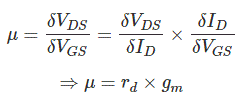Want To Learn Faster? 🎓
Get electrical articles delivered to your inbox every week.
No credit card required—it’s 100% free.